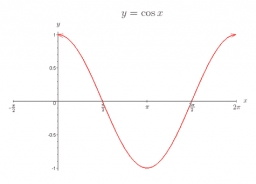# Trigonometric 50551

Solve the trigonometric equation:

cos (x-52°) = 1

x1 =  52 °
x2 =  412 °

### Step-by-step explanation:Did you find an error or inaccuracy? Feel free to write us. Thank you!

Tips to related online calculators
Do you have a linear equation or system of equations and looking for its solution? Or do you have a quadratic equation?